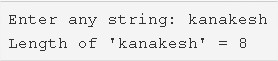# C Program To Find Length Of A String | 4 Simple Ways

C program to find the length of a string – In this article, we will detail in on the means of how to find the length of a string in C programming effectively.

Suitable examples and sample programs have also been added so that you can understand the whole thing very clearly. The compiler has also been added with which you can execute it yourself. In case if you have any doubts related to C program to find the length of the string leave a comment here.

The methods used in this distinct piece are as follows:

• Using Standard Method
• Using Function
• Using Recursion
• Using String Library Function

A string is nothing but an array of characters. The value of a string is determined by the terminating character. Its value is considered to be 0.As you can see in the image uploaded above, firstly, you need to enter any particular string.

As in this case, the string that has been entered is “kanakesh”

We can see that the length of this string is 8 since it has 8 characters.

THus, the numerous ways to do the same in C programming are as follows:

## Using Standard Method – C Program

1. The string is nothing but an array of characters.

2) Read the entered string using gets() function and store into the character array s[].

3) The for loop iterates from the first character to the last character of the array. i.e. the last character of the array is null.

4) Print the i value which is the length of the string.

Output:

### Using Function – Find Length Of A String

1. The main() function calls the stringlength() function by passing string s as an argument.

2) The function stringlength() has the code to find the length of the string.

For loop iterates with the structure for(i=0;s[i]!=’\0′;i++) upto last character of the array. i.e null.

The function stringlength() returns the i value to main() function.The variable i represents the length of the string.

3) The main() function prints the string and length of the string.

Output:

#### Using Recursion

1. The main() calls the recursive function stringlength() by passing string s,i=0 as arguments.

2) The recursive function checks the character at s[i] is null or not. If the character is null then it returns the i value.

a) checks the character at s[i] is null or not. If the character is null then it returns the i value.

b) If the character is null then it returns the i value.

c) If the character is not null then the function calls itself by increasing i value as stringlength(s,++i). The function calls recursively until s[i] is null.

The function calls recursively until s[i] is null.

3) Then the main() function prints the i value which is the string length.

Output:

##### Using String Library Function
1. Here we are using library function strlen() to find the length of the string.The strlen() is available at ‘string.h’ header file.

2) The main() calls the strlen() predefined function by passing string s as an argument. The serlen() calculates the length of the string and the value assigned to the variable length.

3) Print the length value.

Output:

x

## C Program To Check If Alphabet, Digit or Special Character | C Programs

C program to check for an alphabet, digit or special character – In this article, ...